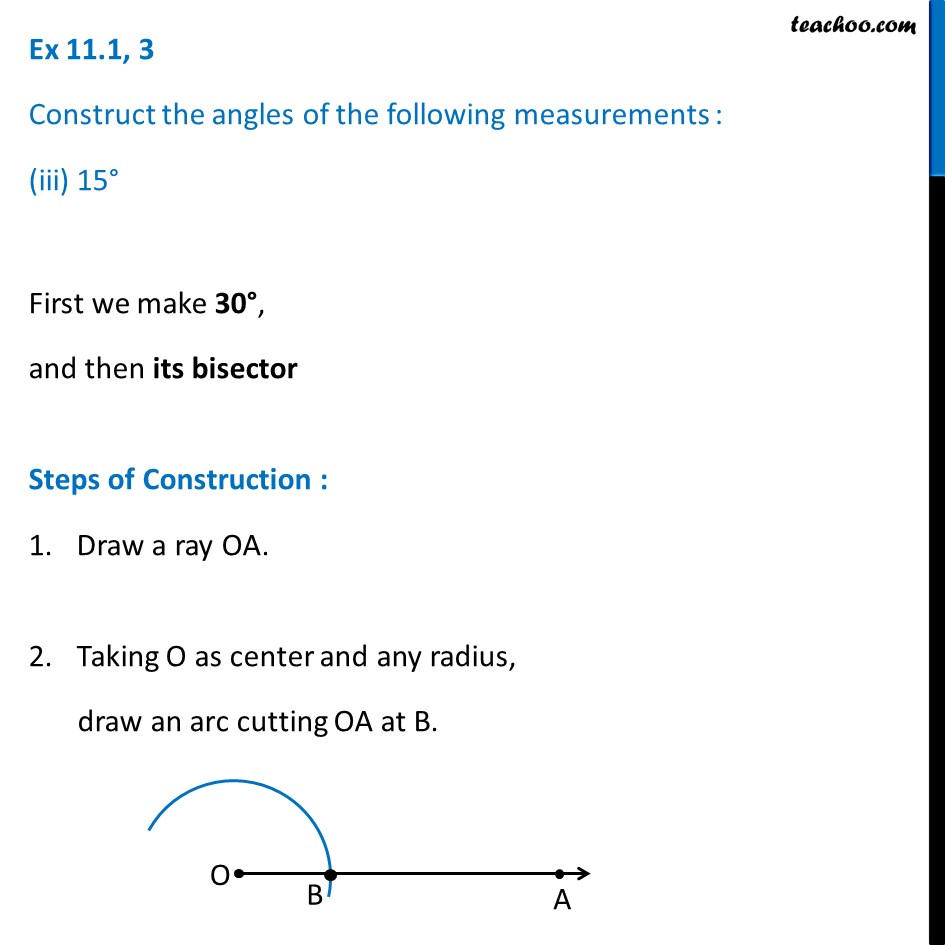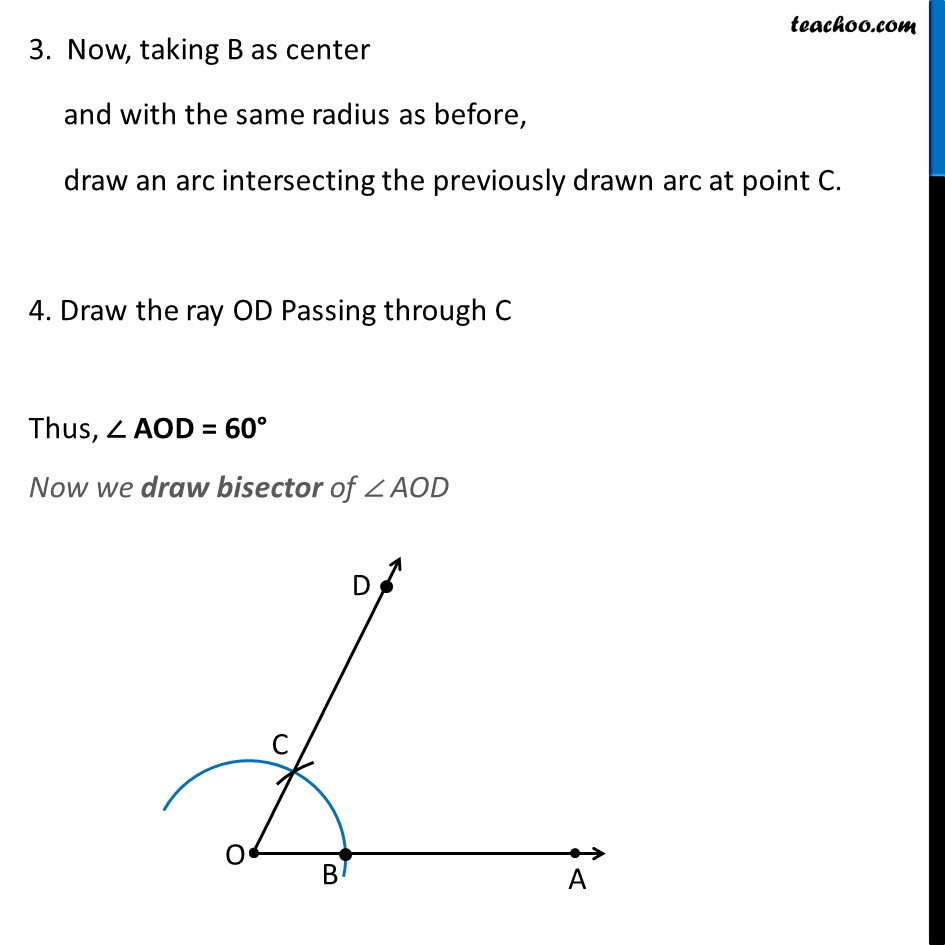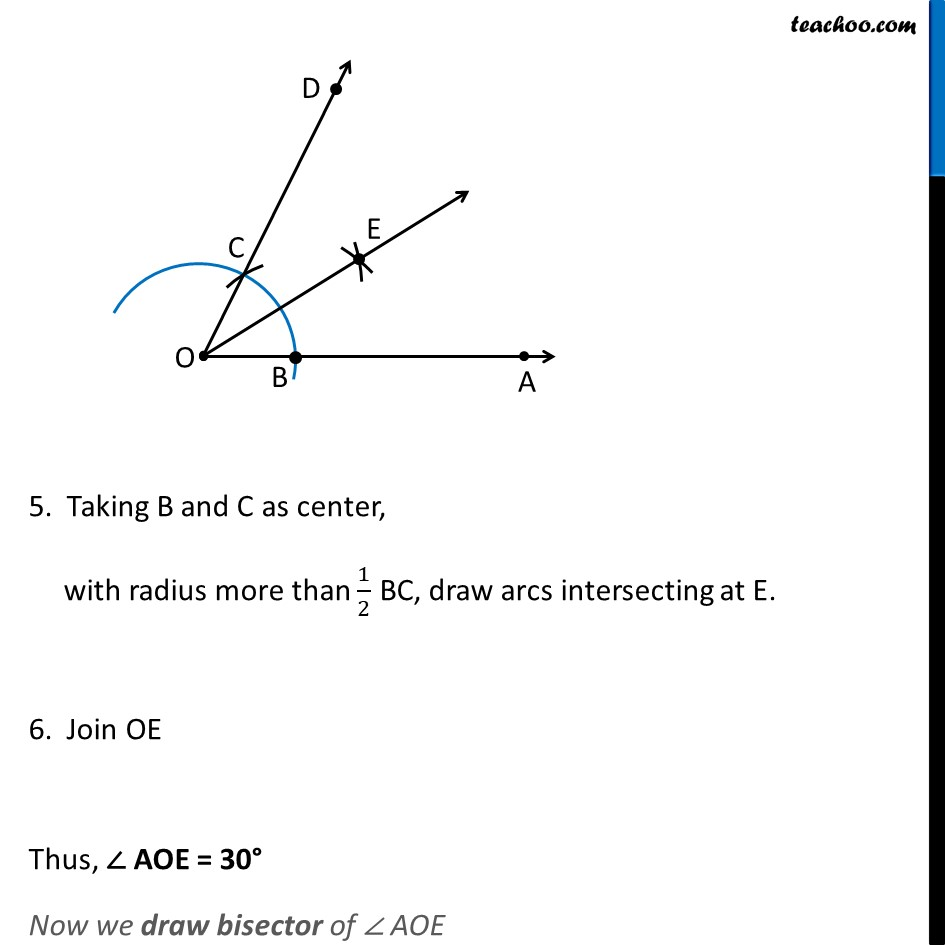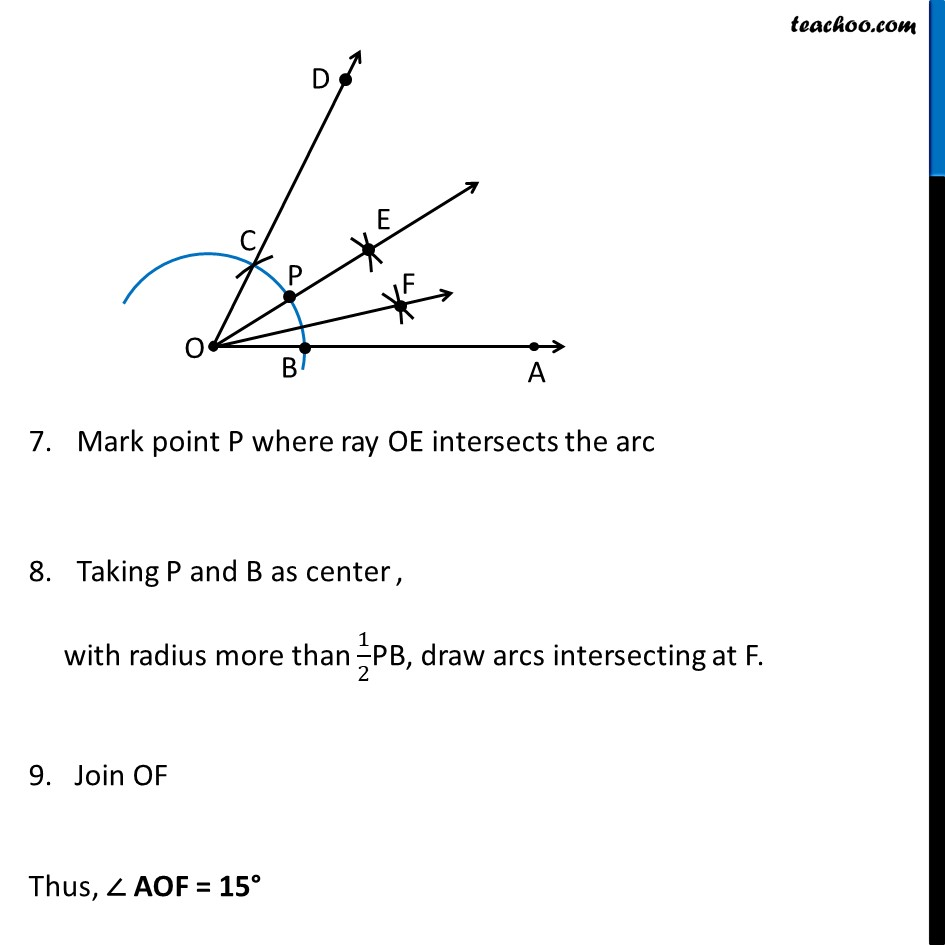1. Chapter 11 Class 9 Constructions (Term 2)
2. Serial order wise
3. Ex 11.1

Transcript

Ex 11.1, 3 Construct the angles of the following measurements : (iii) 15° First we make 30°, and then its bisector Steps of Construction : Draw a ray OA. Taking O as center and any radius, draw an arc cutting OA at B. 3. Now, taking B as center and with the same radius as before, draw an arc intersecting the previously drawn arc at point C. 4. Draw the ray OD Passing through C Thus, ∠ AOD = 60° Now we draw bisector of ∠ AOD 5. Taking B and C as center, with radius more than 1/2 BC, draw arcs intersecting at E. 6. Join OE Thus, ∠ AOE = 30° Now we draw bisector of ∠ AOE Mark point P where ray OE intersects the arc Taking P and B as center , with radius more than 1/2PB, draw arcs intersecting at F. 9. Join OF Thus, ∠ AOF = 15°

Ex 11.1

Chapter 11 Class 9 Constructions (Term 2)
Serial order wise